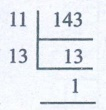Home | | Maths 6th Std | Exercise 1.1

# Exercise 1.1

6th Maths : Term 2 Unit 1 : Numbers : Exercise 1.1 : Text Book Back Exercises Questions with Answers, Solution

Exercise 1.1

1. Fill in the blanks

(i) The number of prime numbers between 11 and 60 is 12.

(ii) The numbers 29 and 31 are twin primes.

(iii) 3753 is divisible by 9 and hence divisible by 3.

(iv) The number of distinct prime factors of the smallest 4 digit number is 2.

(v) The sum of distinct prime factors of 30 is 10 (2 + 3 + 5 = 10).2. Say True or False

(i) The sum of any number of odd numbers is always even. [False]

(ii) Every natural number is either prime or composite. [False]

(iii) If a number is divisible by 6, then it must be divisible by 3. [True]

(iv) 16254 is divisible by each of 2, 3, 6 and 9. [True]

(v) The number of distinct prime factors of 105 is 3. [True]

3. Write the smallest and the biggest two digit prime number.

The smallest prime number = 11

The bigger prime number = 97

4. Write the smallest and the biggest three digit composite number.

The smallest composite number = 100

The biggest composite number = 999

5. The sum of any three odd natural numbers is odd. Justify this statement with an example.

Yes. 5 + 7 + 9 = 21

6. The digits of the prime number 13 can be reversed to get another prime number 31. Find if any such pairs exist upto 100.

(17,71), (37,73), (79,97)

7. Your friend says that every odd number is prime. Give an example to prove him/her wrong.

False. 15 is odd number but not prime number.

8. Each of the composite numbers has atleast three factors. Justify this statement with an example.

True. The composite number 4 has three factors 1, 2 and 4.

9. Find the dates of any month in a calendar which are divisible by both 2 and 3.

The dates in a calendar which are divisible by both 2 and 3 are 6, 12, 18, 24, 30 (Excluding February).

10. I am a two digit prime number and the sum of my digits is 10. I am also one of the factors of 57. Who am I?

Factors of 57 are 1, 3, 19 and 57

Two digit prime number 19

sum of the digits 1 + 9 = 10

11. Find the prime factorisation of each number by factor tree method and division method.

a) 60 b) 128 c) 144 d) 198 e) 420 f) 999

a) 60b) 128c) 144d) 198e) 420Factor tree method : 420 = 2 × 2 × 3 × 5 × 7

Division method : 420 = 2 × 2 × 3 × 5 × 7

f) 999Factor tree method : 999 = 3 × 3 × 3 × 37

Division method : 999 = 3 × 3 × 3 × 37

12. If there are 143 math books to be arranged in equal numbers in all the stacks, then find the number of books in each stack and also the number of stacks.If the number of stacks 11, number of books in each stacks are 13

If the number of stacks 13, number of books in each stacks are 11.

Objective Type Questions

13. The difference between two successive odd numbers is

a) 1

b) 2

c) 3

d) 0

14. The only even prime number is

a) 4

b) 6

c) 2

d) 0

15. Which of the following numbers is not a prime?

a) 53

b) 92

c) 97

d) 71

16. The sum of the factors of 27 is

a) 28

b) 37

c) 40

d) 31

17. The factors of a number are 1, 2, 4, 5, 8, 10, 16, 20, 40 and 80.What is the number?

a) 80

b) 100

c) 128

d) 160

18. The prime factorisation of 60 is 2 ×2 × 3 × 5. Any other number which has the same prime factorisation as 60 is

a) 30

b) 120

c) 90

d) impossible

19. If the number 6354 * 97 is divisible by 9, then the value * is

a) 2

b) 4

c) 6

d) 7

20. The number 87846 is divisible by

a) 2 only

b) 3 only

c) 11 only

d) all of these

Exercise 1.1

1. i) 12 ii) 31 iii) 3 iv) 2 v) 10

2. i) False ii) False iii) True iv) True v) True

3. smallest → 11; biggest → 97

4. smallest → 100; biggest → 999

5. True. 3 + 7 + 9 = 19 is odd

6. (17, 71), (37,73) & (79,97)

7. False. 9 is odd number but not prime

8. True. The composite number 4 has 3 factors namely 1, 2, and 4.

9. 6, 12, 18, 24, 30 (excluding February)

10. 19

11. a) 60 = 2 × 2 × 3 × 5

b) 128 = 2 × 2 × 2 × 2 × 2 × 2 × 2

c) 144 = 2 × 2 × 2 × 2 × 3 × 3

d) 198 = 2 × 3 × 3 × 11

e) 420 = 2 × 2 × 3 × 5 × 7

f) 999 = 3 × 3 × 3 × 37

12. (11, 13) or (13, 11)

Objective Type Questions

13. b) 2

14. c) 2

15. b) 92

16. c) 40

17. a) 80

18. d) impossible

19. a) 2

20. d) all of these

Tags : Questions with Answers, Solution | Numbers | Term 2 Chapter 1 | 6th Maths , 6th Maths : Term 2 Unit 1 : Numbers
Study Material, Lecturing Notes, Assignment, Reference, Wiki description explanation, brief detail
6th Maths : Term 2 Unit 1 : Numbers : Exercise 1.1 | Questions with Answers, Solution | Numbers | Term 2 Chapter 1 | 6th Maths